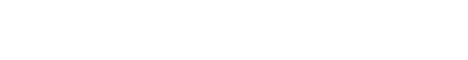# Identify if the acid-base balance is compensated or uncompensatedIf there is any kind of acid-base imbalance occurs, then the homeostasis mechanism of the human body tries to balance it. This can happen if the PCO and HCOwill be in a normal range. So, at first, we will know what are the normal values of PH, PCO2, and HCOions.

P= 7.35- 7.45

PCO2= 35- 45 mm/Hg

HCO3 =22-26 mmol/L

## compensated or uncompensated

To know about the compensation mechanism, let’s take some examples.

## There is a patient having asthma. During ABG analysis we found that the PH value, PCO2, and HCO3 values are  7.30, 50mm/Hg, and 49mmol/L respectively. So, is it acidosis or alkalosis, and identify is it compensated or uncompensated ?

Ans. Here the P=7.30, which means acidosis. then PCO2 =50mm/Hg, which means respiratory acidosis. Then HCO= 49mmol/L, which means metabolic alkalosis. But, here only the value of PCO2 will be considered and don’t give much importance to the value of HCO3. because the body is now acidic as per the value of pH. So, here the HCO3 was tried to balance it. but, It happened partially. not only the value of p or PCO2 but also the asthmatic condition of the patient shows that it is the matter of respiratory acidosis.

## There is a patient having PH, PCO2 and HCO3  are 7.50, 51 mm/Hg, and 41mmol/L respectively. Identify if it is compensated or uncompensated (acidosis/alkalosis)?

Ans.  Here the value of P=7.50, which means alkalosis. The value of PCO2 = 51, which means respiratory acidosis and at last the value of HCO3 =41, which means metabolic alkalosis. But, here we will be considered only the value of HCO3 and don’t focus on the value of PCO2. Because the blood is in the alkaline state as per the value of PH. here also the PCO2 will be tried to compensate but failed. so, It is metabolic alkalosis and there is partial compensation takes place.

## There is a man having PH (7.35).But, the value of PCO2 and HCO3 are 49 mm/Hg, 30 mmol/L respectively.so, what kind of compensation it is?

Ans. The Pis 7.35 which means it is normal. On the other hand the value of PCO2 is 49, which means respiratory acidosis, The value of HCO3 is 30, which means metabolic alkalosis. So, what we can do in this case, we have to divide 7.35-7.45 into two parts. like 7.35 -7.39 is considered as acidic and 7.40-7.45 is consider as basic. In this sense, the pH is 7.35 which means acidosis occurs. As the blood is acidic that’s why we will only consider the pcoand ignore the value of HCO3. so, It is called respiratory acidosis. but, It is fully compensated because of HCO3.

## During ABG analysis, the nurse examined that the pH of the patient is 7.26, PCO2 is 49mm/Hg and the value of HCO3 is 24. So, is it compensated or uncompensated (acidosis/alkalosis)?

Ans. The value of the patient’s PH is 7.26 which means acidosis. as the value of PCO2 is 49, which means respiratory acidosis takes place. and the value of HCO3 is 24 which means metabolic acidosis. So, here we can see that there is no compensation mechanism takes place as the bicarbonate ions didn’t increase in their normal values. It is basically a respiratory acidosis case.

So, that’s all about the compensation mechanism of acid-base balance. To know about the whole concept of the acid-base balance, please read my previous blog which is about the “acid-base balance”.